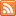Content Tagged “LO 4.21”Linear Relationships – Linear Regression

Published: Dec 24th, 2012

IMPORTANT: The methods covered in this section on linear regression are only applicable for LINEAR relationships.   Summarizing the Pattern of the Data with a Line In General Let’s Summarize CO-4: Distinguish among […]Linear Relationships – Correlation

Published: Aug 21st, 2012

IMPORTANT: The methods covered in this section on correlation are only applicable for LINEAR relationships.   Introduction The Correlation Coefficient — r Interpretation Properties of r CO-4: Distinguish among different measurement scales, […]Scatterplots

Published: Aug 21st, 2012

Creating Scatterplots Interpreting Scatterplots Direction Form Strength A Labeled (or Grouped) Scatterplot Let’s Summarize CO-4: Distinguish among different measurement scales, choose the appropriate descriptive and inferential statistical methods based on these […]Case Q-Q

Published: Aug 21st, 2012

CO-4: Distinguish among different measurement scales, choose the appropriate descriptive and inferential statistical methods based on these distinctions, and interpret the results. LO 4.20: Classify a data analysis situation involving two variables […]Case C-C

Published: Aug 21st, 2012

Two Categorical Variables Contingency Tables Finding Conditional (Row and Column) Percents Let’s Summarize CO-4: Distinguish among different measurement scales, choose the appropriate descriptive and inferential statistical methods based on these distinctions, […]Case C-Q

Published: Aug 21st, 2012

Categorical Explanatory and Quantitative Response Let’s Summarize CO-4: Distinguish among different measurement scales, choose the appropriate descriptive and inferential statistical methods based on these distinctions, and interpret the results. LO 4.20: Classify […]